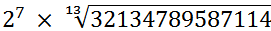2248

《最强大脑》上周玮的3道数学题，应该怎么算？周玮在《最强大脑》上速算的3道数学题，换成是你这样的普通人，要怎么算才能更快一点呢？图片来源：《最强大脑》周玮速算的3道题。

## 最简单的题最需要心算能力

613 =？

613 = ( (63)2)2×6

63是个口算级别的题，对数字敏感的人可以脱口而出216。于是题目接下来变为
(2162)2×6 =？

216= (200+16)×(200+16) = 40000+3200×2+256 = 46656

46656= (46000+656)×(46000+656) = 462×1000000+656×46×2×1000+6562

46= (45+1)×(45+1) = 452+90+1

(10x+5)= x2×102+10x×5×2+5= (x2+x)×102+5= 100x(x+1)+25

45= 4×(4+1)×100+25 = 2025

462= 2025+91 = 2116

656×46×2 = 656×92 = 656×(100-10+2) = 65600-6560+1312 = 60000-960+1312 = 60000+312+40 = 60352

656= (650+6)= 6502+650×6×2+36 = (6×(6+1)×100+25)×100+1300×6+36 = 422500+7800+36 = 430336

46656= 2116000000+60352000+430336 = 2176782336

2176782336×6 = 13060694016

1. 反复对复杂的数字进行以0结尾或者以5结尾的拆分；
2. 利用各类公式来简化计算。

lg(6) = lg(2)+lg(3) = 0.301+0.477 = 0.778

lg(6)×13 =  0.778×13  =10.1

(63)2=4 0000

((63)2)2=16 0000 0000

6×((63)2)2=96 0000 0000≈100 0000 0000

## 看似吓人的开高次方，其实没有那么可怕1391237759766345数字太大，不妨近似一下：lg(3.2) = lg(32*0.1)=lg(32)+lg0.1=lg(2^5)+(-1) = lg(2)×5-1

lg(2)×7+(lg(2)×5-1)/13+1 = 0.3010×7+(0.3010×5-1)/13+1 = 3.147

The End

antares